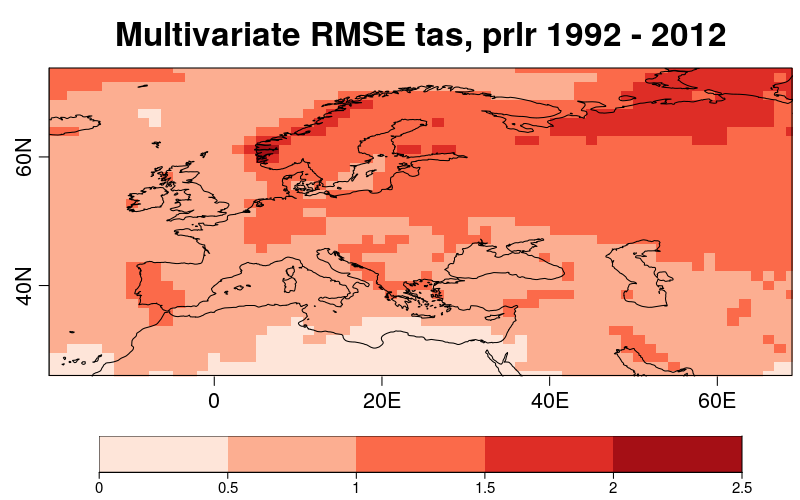Multivariate Root Mean Square Error (RMSE)

To run this vignette, the next R packages should be installed and loaded:

library(s2dverification)
library(RColorBrewer)

Library CSTools, should be installed from CRAN and loaded:

install.packages("CSTools")
library(CSTools)

In this example, the seasonal temperature and precipitation forecasts, initialized in november, will be used to assess the glosea5 seasonal forecasting system from the Met Office, by computing the multivariate RMSE for both temperature and precipitation.

The parameters defined are the initializing month and the variables:

mth = '11'
temp = 'tas'
precip = 'prlr'

The simulations available for this model cover the period 1992-2012. So, the starting and ending dates can be defined by running the following lines:

ini <- 1992
fin <- 2012
start <- as.Date(paste(ini, mth, "01", sep = ""), "%Y%m%d")
end <- as.Date(paste(fin, mth, "01", sep = ""), "%Y%m%d")
dateseq <- format(seq(start, end, by = "year"), "%Y%m%d")

The grid in which all data will be interpolated should be also specified. The observational dataset used in this example is the EraInterim.

grid <- "256x128"
obs <- "erainterim"

Using the CST_Load function from CSTool package, the data available in our data store can be loaded. The following lines show how this function can be used. Here, the data is loaded from a previous saved .RData file: Ask nuria.perez at bsc.es for the data to run the recipe.

require(zeallot)

glosea5 <- list(path = '/esnas/exp/glosea5/specs-seasonal_i1p1/\$STORE_FREQ\$_mean/\$VAR_NAME\$-allmemb/\$VAR_NAME\$_\$START_DATE\$.nc')

c(exp_T, obs_T) %<-%
CST_Load(var = temp, exp = list(glosea5),
obs = obs, sdates = dateseq, leadtimemin = 2, leadtimemax = 4,
latmin = 25, latmax = 75, lonmin = -20, lonmax = 70, output = 'lonlat',
nprocs = 1, storefreq = "monthly", sampleperiod = 1, nmember = 9,
method = "bilinear", grid = paste("r", grid, sep = ""))

c(exp_P, obs_P) %<-%
CST_Load(var = precip,  exp = list(glosea5),
obs = obs, sdates = dateseq, leadtimemin = 2, leadtimemax = 4,
latmin = 25, latmax = 75, lonmin = -20, lonmax = 70, output = 'lonlat',
nprocs = 1, storefreq = "monthly", sampleperiod = 1, nmember = 9,
method = "bilinear", grid = paste("r", grid, sep = ""))
#save(exp_T, obs_T, exp_P, obs_P, file = "./tas_prlr_toydata.RData")

# Or use the following line to load the file provided in .RData format:

There should be four new elements loaded in the R working environment: exp_T, obs_T, exp_P and obs_P. The first two elements correspond to the experimental and observed data for temperature and the other are the equivalent for the precipitation data. It's possible to check that they are of class sd2v_cube by running:

class(exp_T)
class(obs_T)
class(exp_P)
class(obs_P)

Loading the data using CST_Load allows to obtain two lists, one for the experimental data and another for the observe data, with the same elements and compatible dimensions of the data element:

> dim(exp_T\$data)
dataset  member   sdate   ftime     lat     lon
1       9      21       3      35      64
> dim(obs_T\$data)
dataset  member   sdate   ftime     lat     lon
1       1      21       3      35      64

Latitudes and longitudes of the common grid can be saved:

Lat <- exp_T\$lat
Lon <- exp_T\$lon

The next step is to compute the anomalies of the experimental and observational data using CST_Anomaly function, which could be applied over data from each variable, and in this case it's compute applying cross validation technique over individual members:

c(ano_exp_T, ano_obs_T) %<-% CST_Anomaly(exp = exp_T, obs = obs_T, cross = TRUE, memb = TRUE)
c(ano_exp_P, ano_obs_P) %<-% CST_Anomaly(exp = exp_P, obs = obs_P, cross = TRUE, memb = TRUE)

The original dimensions are preserved and the anomalies are stored in the data element of the correspondent object:

> str(ano_exp_T\$data)
num [1, 1:9, 1:21, 1:3, 1:35, 1:64] -1.647 1.575 2.77 0.048 -1.886 ...
- attr(*, "dimensions")= chr [1:6] "dataset" "member" "sdate" "ftime" ...
> str(ano_obs_T\$data)
num [1, 1, 1:21, 1:3, 1:35, 1:64] 0.0235 1.546 1.3885 -0.344 -5.972 ...
- attr(*, "dimensions")= chr [1:6] "dataset" "member" "sdate" "ftime" ...

Two lists containing the experiment ,ano_exp, and the observation, ano_obs, lists should be put together to serve as input of the function to compute multivariate RMSEs.

Furthermore, some weights can be applied to the difference variables based on their relative importance (if no weights are given, a value of 1 is automatically assigned to each variable). For this example, we'll give a weight of 2 to the temperature dataset and a weight of 1 to the precipitation dataset:

ano_exp <- list(ano_exp_T, ano_exp_P)
ano_obs <- list(ano_obs_T, ano_obs_P)
weight <- c(2, 1)

2.- Computing and plotting multivariate RMSEs

The multivariate RMSE gives an indication of the forecast performance (RMSE) for multiple variables simultaneously. Variables can be weighted based on their relative importance. It is obtained by running the CST_MultivarRMSE function:

mvrmse <- CST_MultivarRMSE(exp = ano_exp, obs = ano_obs, weight)

The function CST_MultivarRMSE returns the multivariate RMSE value for 2 or more variables. The output is a CSTool object containing the RMSE values in the data element and other relevant information:

> class(mvrmse)
> str(mvrmse\$data)
num [1, 1, 1, 1:35, 1:64] 0.764 0.8 0.67 0.662 0.615 ...
> str(mvrmse\$Variable)
Named chr [1:2] "tas" "prlr"
- attr(*, "names")= chr [1:2] "varName" "varName"

The following lines plot the multivariate RMSE

PlotEquiMap(mvrmse\$data, lon = Lon, lat = Lat, filled.continents = FALSE,
toptitle = "Multivariate RMSE tas, prlr 1992 - 2012", colNA = "white",
bar_limits = c(0,2.5), cols = brewer.pal(n=5,name='Reds'),
fileout = "./MultivarRMSE_gloseas5_tas_prlr_1992-2012.png")